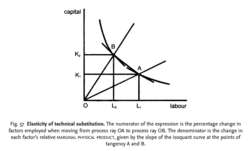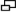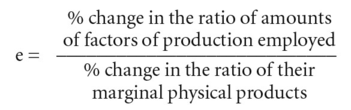# elasticity of technical substitutionFig. 57 Elasticity of technical substitution. The numerator of the expression is the percentage change in factors employed when moving from process ray OA to process ray OB. The denominator is the change in each factor's relative MARGINAL PHYSICAL PRODUCT, given by the slope of the isoquant curve at the points of tangency A and B.

## elasticity of technical substitution

the rate at which one FACTOR INPUT can be substituted for another with OUTPUT remaining constant. For example, the rate of substitution between CAPITAL and LABOUR may be expressed as:The denominator in the above expression is known as the MARGINAL RATE OF TECHNICAL SUBSTITUTION. Essentially, the elasticity of substitution is the change in factor proportions used (the numerator) in relation to their substitutability (the denominator). The above expressions may be illustrated graphically using ISOQUANT CURVES (equal product curves) and PROCESS RAYS.

The elasticity of substitution between factor inputs is not infinite. Where no substitution is possible (e = 0), inputs must be used in fixed proportions; where factors are perfect substitutes, e = ∞. The actual measure will lie somewhere between the two. Where it is one (as exhibited in the COBB- DOUGLAS PRODUCTION FUNCTION), there exist constant returns to scale, i.e. labour can be substituted for capital in any given proportions, and vice-versa, without affecting output.

Site: Follow: Share:
Open / Close